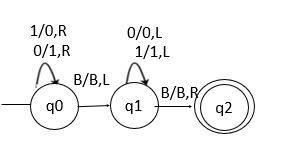# Draw a Turing machine to find 1’s complement of a binary number

1’s complement means transforming the 0 bit to 1 and the 1 bit to 0.

Let the input be −

 B 0 0 1 0 1 1 1 0 B

The output is as follows −

 B 1 1 0 1 0 0 0 1 B

## Concept

The concept is explained below −

Step 1 − Start scanning the input from left to right.

Step 2 − If the R/W is at 1, then make it 0 and move right.

Step 3 − If the R/W is at 0, then make it 1 and move right.

Step 4 − Repeat the steps given above and we will reach B (blank).

Step 5 − Then move the R/W head all the way to the left without changing anything until it reaches the first character.

Step 6 − The string will be accepted here.

The Turing machine which implements the above-mentioned steps is given below. Here, Q0 is the initial state and Q2 is the final state.## Explanation

• q0 state is to replace ‘1’ with ‘0’ and ‘0’ with ‘1’ and it moves to the right, whenever the BLANK is reached, moves towards the left.

• Using state ‘q2’ we reach the start of the string, whenever the BLANK is reached, move towards right and reach the final state q2.